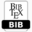## Statistical Characteristic of Ratio and Product of Rician Random Variables and its Application in Analysis of Wireless Communication Systems

 AUTHOR(S): Dragana Krstić, Mihajlo Stefanović, Zoran Jovanović, Radmila Gerov, Vladeta Milenković TITLE Statistical Characteristic of Ratio and Product of Rician Random Variables and its Application in Analysis of Wireless Communication SystemsPDFBIBTEX ABSTRACT In this paper, the product of two Rician random variables, the ratio of two Rician random variables, the ratio of Rician random variable and product of two Rician random variables, and the ratio of product of two Rician random variables and Rician random variable are considered. The expressions for the level crossing rate of product of two Rician random processes, ratio of two Rician random processes, ratio of Rician random process and product of two Rician random processes, and ratio of product of two Rician random processes and Rician random process are derived. We use those results for calculation the average fade duration of wireless communication system operating over Rician multipath fading channels. The influence of Rician factor K on the level crossing rate is analyzed and discussed. KEYWORDS Rician random variable, product, ratio, wireless communication systems. REFERENCES  N. Zlatanov, Z. Hadzi-Velkov, G. K. Karagiannidis, Level Crossing Rate and Average Fade Duration of the Double Nakagami-m Random Process and Application in MIMO Keyhole Fading Channels, IEEE Communications Letters, Vol. 12, Issue 11, November 2008, pp. 822 – 824.  N. Zlatanov, Z. Hadzi-Velkov, G. K. Karagiannidis, Level Crossing Rate and Average Fade Duration of the Double Nakagami-m Random Process and Application in MIMO Keyhole Fading Channels, IEEE Communications Letters, Vol. 12, Issue 11, November 2008, pp. 822 – 824.  Z. Hadzi-Velkov, N. Zlatanov, G. K. Karagiannidis, On the second order statistics of the multihop Rayleigh fading channel, IEEE Transactions on Communications, Vol. 57, Issue: 6, June 2009, pp. 1815 – 1823  A. Matović, E. Mekić, N. Sekulović, M. Stefanović, M. Matović, Ĉ Stefanović, The Distribution of the Ratio of the Products of Two Independent - Variates and Its Application in the Performance Analysis of Relaying Communication Systems, Mathematical Problems in Engineering, Vol. 2013, Article ID 147106, 6 pages, 2013.  E. Mekić, M. Stefanović, P. Spalević, N. Sekulović, A. Stanković, “Statistical analysis of ratio of random variables and its application in performance analysis of multi-hop wireless transmissions”, Mathematical Problems in Engineering, Vol. 2012, Article ID 841092  A. Stanković, N. Sekulović, M. Stefanović, E. Mekić, S. Zdravković, “Statistical characteristic of ratio of product of two random Rayleigh variables and Rayleigh random variable and its application in performance analysis of wireless communication systems”, Proc. of InfotehJahorina, Istoĉno Sarajevo, BIH, 16-28 March 2011, vol. 10, Ref. B-I-3, pp.79-81.  D. H. Pavlović, N. M. Sekulović, G. V. Milovanović, A. S. Panajotović, M. Ĉ. Stefanović, Z. J. Popović, Statistics for Ratios of Rayleigh, Rician, Nakagami-𝑚, and Weibull Distributed Random Variables, Mathematical Problems in Engineering, Vol. 2013, Article ID 252804, http://dx.doi.org/10.1155/2013/252804  M. K. Simon, M. S. Alouini, Digital Communication over Fading Channels, USA: John Wiley & Sons. 2000.  W.C.Y. Lee, Mobile communications engineering, Mc-Graw-Hill, NewYork, USA, 2003. Cite this paper Dragana Krstić, Mihajlo Stefanović, Zoran Jovanović, Radmila Gerov, Vladeta Milenković. (2016) Statistical Characteristic of Ratio and Product of Rician Random Variables and its Application in Analysis of Wireless Communication Systems. International Journal of Mathematical and Computational Methods, 1, 79-86Copyright © 2016 Author(s) retain the copyright of this article.This article is published under the terms of the Creative Commons Attribution License 4.0